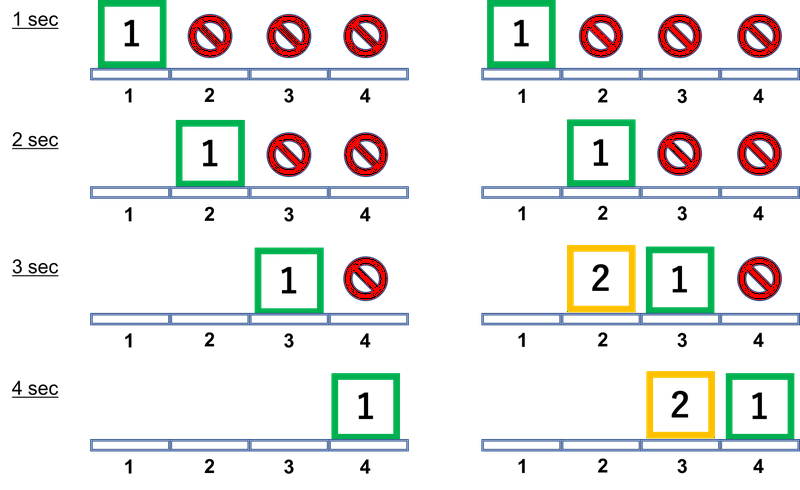A - Window

Time Limit: 2 sec / Memory Limit: 512 MB

### Problem Statement

In the building of Jewelry Art Gallery (JAG), there is a long corridor in the east-west direction. There is a window on the north side of the corridor, and $N$ windowpanes are attached to this window. The width of each windowpane is $W$, and the height is $H$. The $i$-th windowpane from the west covers the horizontal range between $W \times (i - 1)$ and $W \times i$ from the west edge of the window.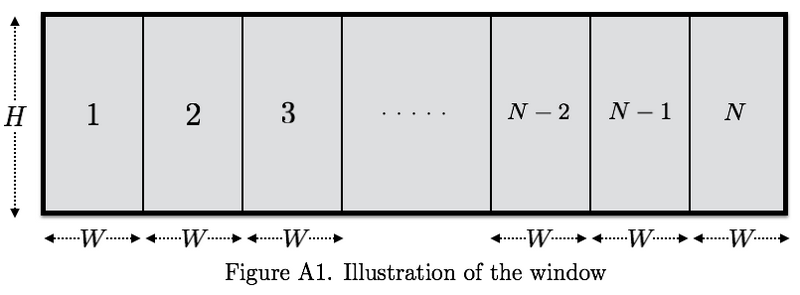You received instructions from the manager of JAG about how to slide the windowpanes. These instructions consist of $N$ integers $x_1, x_2, \dots, x_N$, and $x_i \le W$ is satisfied for all $i$. For the $i$-th windowpane, if $i$ is odd, you have to slide $i$-th windowpane to the east by $x_i$, otherwise, you have to slide $i$-th windowpane to the west by $x_i$.

You can assume that the windowpanes will not collide each other even if you slide windowpanes according to the instructions. In more detail, $N$ windowpanes are alternately mounted on two rails. That is, the $i$-th windowpane is attached to the inner rail of the building if $i$ is odd, otherwise, it is attached to the outer rail of the building.

Before you execute the instructions, you decide to obtain the area where the window is open after the instructions.

### Input

The input consists of a single test case in the format below.

$N$ $H$ $W$ $x_1$ $\cdots$ $x_N$

The first line consists of three integers $N$, $H$, and $W$ ($1 \le N \le 100$, $1 \le H, W \le 100$). It is guaranteed that $N$ is even. The following line consists of $N$ integers $x_1$, $\ldots$, $x_N$ while represent the instructions from the manager of JAG. $x_i$ represents the distance to slide the $i$-th windowpane ($0 \le x_i \le W$).

### Output

Print the area where the window is open after the instructions in one line.

### Sample Input 1

4 3 3
1 1 2 3


### Output for Sample Input 1

9


### Sample Input 2

8 10 18
2 12 16 14 18 4 17 16


### Output for Sample Input 2

370


### Sample Input 3

6 2 2
0 2 2 2 2 0


### Output for Sample Input 3

8


### Sample Input 4

4 1 4
3 3 2 2


### Output for Sample Input 4

6


### Sample Input 5

8 7 15
5 0 9 14 0 4 4 15


### Output for Sample Input 5

189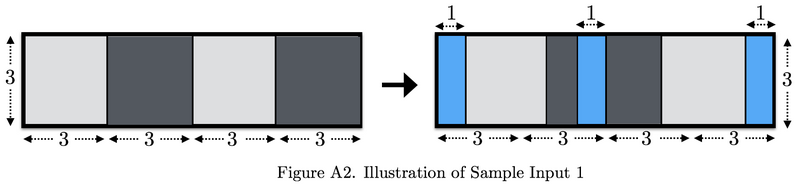B - Tournament Chart

Time Limit: 2 sec / Memory Limit: 512 MB

### Problem Statement

In 21XX, an annual programming contest, Japan Algorithmist GrandPrix (JAG) has become one of the most popular mind sports events.

JAG is conducted as a knockout tournament. This year, $N$ contestants will compete in JAG. A tournament chart is represented as a string. [[a-b]-[c-d]] is an easy example. In this case, there are 4 contestants named a, b, c, and d, and all matches are described as follows:

• Match 1 is the match between a and b.
• Match 2 is the match between c and d.
• Match 3 is the match between [the winner of match 1] and [the winner of match 2].

More precisely, the tournament chart satisfies the following BNF:

• <winner> ::= <person> | "[" <winner> "-" <winner> "]"
• <person> ::= "a" | "b" | "c" | ... | "z"

You, the chairperson of JAG, are planning to announce the results of this year's JAG competition. However, you made a mistake and lost the results of all the matches. Fortunately, you found the tournament chart that was printed before all of the matches of the tournament. Of course, it does not contains results at all. Therefore, you asked every contestant for the number of wins in the tournament, and got $N$ pieces of information in the form of "The contestant $a_i$ won $v_i$ times".

Now, your job is to determine whether all of these replies can be true.

### Input

The input consists of a single test case in the format below.

$S$ $a_1$ $v_1$ $\vdots$ $a_N$ $v_N$

$S$ represents the tournament chart. $S$ satisfies the above BNF. The following $N$ lines represent the information of the number of wins. The $(i+1)$-th line consists of a lowercase letter $a_i$ and a non-negative integer $v_i$ ($v_i \le 26)$ separated by a space, and this means that the contestant $a_i$ won $v_i$ times. Note that $N$ ($2 \le N \le 26$) means that the number of contestants and it can be identified by string $S$. You can assume that each letter $a_i$ is distinct. It is guaranteed that $S$ contains each $a_i$ exactly once and doesn't contain any other lowercase letters.

### Output

Print Yes in one line if replies are all valid for the tournament chart. Otherwise, print No in one line.

### Sample Input 1

[[m-y]-[a-o]]
o 0
a 1
y 2
m 0


### Output for Sample Input 1

Yes


### Sample Input 2

[[r-i]-[m-e]]
e 0
r 1
i 1
m 2


### Output for Sample Input 2

No


C - Prime-Factor Prime

Time Limit: 2 sec / Memory Limit: 512 MB

### Problem Statement

A positive integer is called a "prime-factor prime" when the number of its prime factors is prime. For example, $12$ is a prime-factor prime because the number of prime factors of $12 = 2 \times 2 \times 3$ is $3$, which is prime. On the other hand, $210$ is not a prime-factor prime because the number of prime factors of $210 = 2 \times 3 \times 5 \times 7$ is $4$, which is a composite number.

In this problem, you are given an integer interval $[l, r]$. Your task is to write a program which counts the number of prime-factor prime numbers in the interval, i.e. the number of prime-factor prime numbers between $l$ and $r$, inclusive.

### Input

The input consists of a single test case formatted as follows.

$l$ $r$

A line contains two integers $l$ and $r$ ($1 \le l \le r \le 10^9$), which presents an integer interval $[l, r]$. You can assume that $0 \le r - l < 1{,}000{,}000$.

### Output

Print the number of prime-factor prime numbers in $[l, r]$.

### Sample Input 1

1 9


### Output for Sample Input 1

4


### Sample Input 2

10 20


### Output for Sample Input 2

6


### Sample Input 3

575 57577


### Output for Sample Input 3

36172


### Sample Input 4

180 180


### Output for Sample Input 4

1


### Sample Input 5

9900001 10000000


### Output for Sample Input 5

60997


### Sample Input 6

999000001 1000000000


### Output for Sample Input 6

592955


### Note

In the first example, there are 4 prime-factor primes in $[l, r]$: $4$, $6$, $8$, and $9$.

D - Revenge of the Broken Door

Time Limit: 10 sec / Memory Limit: 512 MB

### Problem Statement

The JAG Kingdom consists of $N$ cities and $M$ bidirectional roads. The $i$-th road $(u_i,v_i,c_i)$ connects the city $u_i$ and the city $v_i$ with the length $c_i$. One day, you, a citizen of the JAG Kingdom, decided to go to the city $T$ from the city $S$. However, you know that one of the roads in the JAG Kingdom is currently under construction and you cannot pass the road. You don't know which road it is. You can know whether a road is under construction only when you are in either city connected by the road.

Your task is to minimize the total length of the route in the worst case. You don't need to decide a route in advance of departure and you can choose where to go next at any time. If you cannot reach the city $T$ in the worst case, output -1.

### Input

The input consists of a single test case formatted as follows.

$N$ $M$ $S$ $T$ $u_1$ $v_1$ $c_1$ $\vdots$ $u_M$ $v_M$ $c_M$

The first line contains four integers $N$, $M$, $S$, and $T$, where $N$ is the number of the cities ($2 \leq N \leq 100{,}000$), $M$ is the number of the bidirectional roads ($1 \leq M \leq 200{,}000$), $S$ is the city you start from ($1 \le S \le N$), and $T$ is the city you want to reach to ($1 \le T \le N$, $S \neq T$). The following $M$ lines represent road information: the $i$-th line of the $M$ lines consists of three integers $u_i$, $v_i$, $c_i$, which means the $i$-th road connects the cities $u_i$ and $v_i$ ($1 \leq u_i, v_i, \leq N$, $u_i \neq v_i$) with the length $c_i$ ($1 \leq c_i \leq 10^9$). You can assume that all the pairs of the cities are connected if no road is under construction. That is, there is at least one route from city $x$ to city $y$ with given roads, for all cities $x$ and $y$. It is also guaranteed that there are no multiple-edges, i.e., $\{u_i, v_i\} \neq \{u_j, v_j\}$ for all $1 \le i < j \le M$.

### Output

Output the minimum total length of the route in the worst case. If you cannot reach the city $T$ in the worst case, output -1.

### Sample Input 1

3 3 1 3
1 2 1
2 3 5
1 3 3


### Output for Sample Input 1

6


### Sample Input 2

4 4 1 4
1 2 1
2 4 1
1 3 1
3 4 1


### Output for Sample Input 2

4


### Sample Input 3

5 4 4 1
1 2 3
2 3 4
3 4 5
4 5 6


### Output for Sample Input 3

-1


E - Tree Separator

Time Limit: 2 sec / Memory Limit: 512 MB

### Problem Statement

You are given a tree $T$ and an integer $K$. You can choose arbitrary distinct two vertices $u$ and $v$ on $T$. Let $P$ be the simple path between $u$ and $v$. Then, remove vertices in $P$, and edges such that one or both of its end vertices is in $P$ from $T$. Your task is to choose $u$ and $v$ to maximize the number of connected components with $K$ or more vertices of $T$ after that operation.

### Input

The input consists of a single test case formatted as follows.

$N \ K$ $u_1 \ v_1$ $\vdots$ $u_{N-1} \ v_{N-1}$

The first line consists of two integers $N, K \ (2 \le N \le 100{,}000, 1 \le K \le N)$. The following $N-1$ lines represent the information of edges. The $(i+1)$-th line consists of two integers $u_i, v_i \ (1 \le u_i, v_i \le N \text{ and } u_i \ne v_i \text{ for each } i)$. Each $\{u_i, v_i\}$ is an edge of $T$. It's guaranteed that these edges form a tree.

### Output

Print the maximum number of connected components with $K$ or more vertices in one line.

### Sample Input 1

2 1
1 2


### Output for Sample Input 1

0


### Sample Input 2

7 3
1 2
2 3
3 4
4 5
5 6
6 7


### Output for Sample Input 2

1


### Sample Input 3

12 2
1 2
2 3
3 4
4 5
3 6
6 7
7 8
8 9
6 10
10 11
11 12


### Output for Sample Input 3

4


### Sample Input 4

3 1
1 2
2 3


### Output for Sample Input 4

1


### Sample Input 5

3 2
1 2
2 3


### Output for Sample Input 5

0


### Sample Input 6

9 3
1 2
1 3
1 4
4 5
4 6
4 7
7 8
7 9


### Output for Sample Input 6

2


F - RPG Maker

Time Limit: 2 sec / Memory Limit: 512 MB

### Problem Statement

You are planning to create a map of an RPG. This map is represented by a grid whose size is $H \times W$. Each cell in this grid is either @, *, #, or .. The meanings of the symbols are as follows.

• @: The start cell. The story should start from this cell.
• *: A city cell. The story goes through or ends with this cell.
• #: A road cell.
• .: An empty cell.

You have already located the start cell and all city cells under some constraints described in the input section, but no road cells have been located yet. Then, you should decide which cells to set as road cells.

Here, you want a "journey" exists on this map. Because you want to remove the branch of the story, the journey has to be unforked. More formally, the journey is a sequence of cells and must satisfy the following conditions:

1. The journey must contain as many city cells as possible.
2. The journey must consist of distinct non-empty cells in this map.
3. The journey must begin with the start cell.
4. The journey must end with one of the city cells.
5. The journey must contain all road cells. That is, road cells not included in the journey must not exist.
6. The journey must be unforked. In more detail, all road cells and city cells except for a cell at the end of the journey must share edges with the other two cells both of which are also contained in the journey. Then, each of the start cell and a cell at the end of the journey must share an edge with another cell contained in the journey.
7. You do not have to consider the order of the cities to visit during the journey.

Initially, the map contains no road cells. You can change any empty cells to road cells to make a journey satisfying the conditions above. Your task is to print a map which maximizes the number of cities in the journey.

### Input

The input consists of a single test case of the following form.

$H$ $W$ $S_1$ $S_2$ $\vdots$ $S_H$

The first line consists of two integers $H$ and $W$. $H$ and $W$ are guaranteed to satisfy $H = 4n - 1$ and $W = 4m - 1$ for some positive integers $n$ and $m$ ($1 \le n, m \le 10$). The following $H$ lines represent a map without road cells. The $(i+1)$-th line consists of a string $S_i$ of length $W$. The $j$-th character of $S_i$ is either *, @ or . if both $i$ and $j$ are odd, otherwise .. The number of occurrences of @ in the grid is exactly one. It is guaranteed that there are one or more city cells on the grid.

### Output

Print a map indicating a journey. If several maps satisfy the condition, you can print any of them.

### Sample Input 1

11 7
.......
.......
*.....*
.......
..@....
.......
*......
.......
....*..
.......
.......


### Output for Sample Input 1

.......
.......
*#####*
......#
..@...#
..#.###
*##.#..
#...#..
####*..
.......
.......


### Sample Input 2

7 11
........*..
...........
...........
...........
....*...*..
...........
..*.@...*..


### Output for Sample Input 2

........*..
........#..
........#..
........#..
..##*##.*..
..#...#.#..
..*#@.##*..


G - Coin Slider

Time Limit: 2 sec / Memory Limit: 512 MB

### Problem Statement

You are playing a coin puzzle. The rule of this puzzle is as follows:

There are $N$ coins on a table. The $i$-th coin is a circle with $r_i$ radius, and its center is initially placed at $(sx_i, sy_i)$. Each coin also has a target position: you should move the $i$-th coin so that its center is at $(tx_i, ty_i)$. You can move coins one by one and can move each coin at most once. When you move a coin, it must move from its initial position to its target position along the straight line. In addition, coins cannot collide with each other, including in the middle of moves.

The score of the puzzle is the number of the coins you move from their initial position to their target position. Your task is to write a program that determines the maximum score for a given puzzle instance.

### Input

The input consists of a single test case formatted as follows.

$N$ $r_{1}$ $sx_{1}$ $sy_{1}$ $tx_{1}$ $ty_{1}$ $\vdots$ $r_{N}$ $sx_{N}$ $sy_{N}$ $tx_{N}$ $ty_{N}$

The first line contains an integer $N$ ($1 \le N \le 16$), which is the number of coins used in the puzzle. The $i$-th line of the following $N$ lines consists of five integers: $r_i$, $sx_i$, $sy_i$, $tx_i$, and $ty_i$ ($1 \le r_i \le 1{,}000$, $-1{,}000 \le sx_i, sy_i, tx_i, ty_i \le 1{,}000$, $(sx_i, sy_i) \neq (tx_i, ty_i)$). They describe the information of the $i$-th coin: $r_i$ is the radius of the $i$-th coin, $(sx_i, sy_i)$ is the initial position of the $i$-th coin, and $(tx_i, ty_i)$ is the target position of the $i$-th coin.

You can assume that the coins do not contact or are not overlapped with each other at the initial positions. You can also assume that the maximum score does not change even if the radius of each coin changes by $10^{-5}$.

### Output

Print the maximum score of the given puzzle instance in a line.

### Sample Input 1

3
2 0 0 1 0
2 0 5 1 5
4 1 -10 -5 10


### Output for Sample Input 1

2


### Sample Input 2

3
1 0 0 5 0
1 0 5 0 0
1 5 5 0 5


### Output for Sample Input 2

3


### Sample Input 3

4
1 0 0 5 0
1 0 5 0 0
1 5 5 0 5
1 5 0 0 0


### Output for Sample Input 3

0


### Note

In the first example, the third coin cannot move because it is disturbed by the other two coins.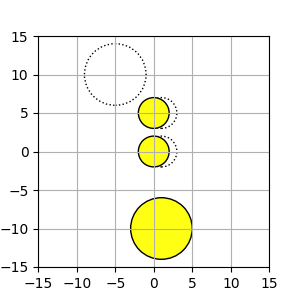Example 1. Initial Positions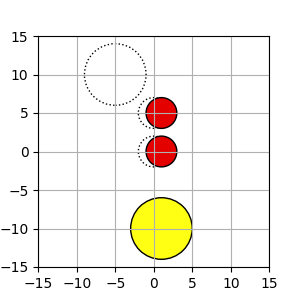Example 1. After Movements

H - Separate String

Time Limit: 2 sec / Memory Limit: 512 MB

### Problem Statement

You are given a string $t$ and a set $S$ of $N$ different strings. You need to separate $t$ such that each part is included in $S$.

For example, the following 4 separation methods satisfy the condition when $t = abab$ and $S = \{a, ab, b\}$.

• $a, b, a, b$
• $a, b, ab$
• $ab, a, b$
• $ab, ab$

Your task is to count the number of ways to separate $t$. Because the result can be large, you should output the remainder divided by $1{,}000{,}000{,}007$.

### Input

The input consists of a single test case formatted as follows.

$N$ $s_1$ $\vdots$ $s_N$ $t$

The first line consists of an integer $N \ (1 \le N \le 100{,}000)$ which is the number of the elements of $S$. The following $N$ lines consist of $N$ distinct strings separated by line breaks. The $i$-th string $s_i$ represents the $i$-th element of $S$. $s_i$ consists of lowercase letters and the length is between $1$ and $100{,}000$, inclusive. The summation of length of $s_{i} \ (1 \le i \le N)$ is at most $200{,}000$. The next line consists of a string $t$ which consists of lowercase letters and represents the string to be separated and the length is between $1$ and $100{,}000$, inclusive.

### Output

Calculate the number of ways to separate $t$ and print the remainder divided by $1{,}000{,}000{,}007$.

### Sample Input 1

3
a
b
ab
abab


### Output for Sample Input 1

4


### Sample Input 2

3
a
b
c
xyz


### Output for Sample Input 2

0


### Sample Input 3

7
abc
ab
bc
a
b
c
aa
aaabcbccababbc


### Output for Sample Input 3

160


### Sample Input 4

10
a
aa
aaa
aaaa
aaaaa
aaaaaa
aaaaaaa
aaaaaaaa
aaaaaaaaa
aaaaaaaaaa
aaaaaaaaaaaaaaaaaaaaaaaaaaaaaaaaaaaaaaaa


### Output for Sample Input 4

461695029


I - Revenge of the Endless BFS

Time Limit: 2 sec / Memory Limit: 512 MB

### Problem Statement

Mr. Endo wanted to write the code that performs breadth-first search (BFS), which is a search algorithm to explore all vertices on a directed graph. An example of pseudo code of BFS is as follows:

1: $current \leftarrow \{start\_vertex\}$ 2: $visited \leftarrow current$ 3: while $visited \ne$ the set of all the vertices 4: $found \leftarrow \{\}$ 5: for $u$ in $current$ 6: for each $v$ such that there is an edge from $u$ to $v$ 7: $found \leftarrow found \cup \{v\}$ 8: $current \leftarrow found \setminus visited$ 9: $visited \leftarrow visited \cup found$

However, Mr. Endo apparently forgot to manage visited vertices in his code. More precisely, he wrote the following code:

1: $current \leftarrow \{start\_vertex\}$ 2: while $current \ne$ the set of all the vertices 3: $found \leftarrow \{\}$ 4: for $u$ in $current$ 5: for each $v$ such that there is an edge from $u$ to $v$ 6: $found \leftarrow found \cup \{v\}$ 7: $current \leftarrow found$

You may notice that for some graphs, Mr. Endo's program will not stop because it keeps running infinitely. Notice that it does not necessarily mean the program cannot explore all the vertices within finite steps. Your task here is to make a program that determines whether Mr. Endo's program will stop within finite steps for a given directed graph in order to point out the bug to him. Also, calculate the minimum number of loop iterations required for the program to stop if it is finite. Since the answer might be huge, thus print the answer modulo $10^9 + 7$, which is a prime number.

### Input

The input consists of a single test case formatted as follows.

$N$ $M$ $u_{1}$ $v_{1}$ $\vdots$ $u_{M}$ $v_{M}$

The first line consists of two integers $N$ ($2 \le N \le 500$) and $M$ ($1 \le M \le 200{,}000$), where $N$ is the number of vertices and $M$ is the number of edges in a given directed graph, respectively. The $i$-th line of the following $M$ lines consists of two integers $u_{i}$ and $v_{i}$ ($1 \le u_{i}, v_{i} \le N$), which means there is an edge from $u_{i}$ to $v_{i}$ in the given graph. The vertex $1$ is the start vertex, i.e. $start\_vertex$ in the pseudo codes. You can assume that the given graph also meets the following conditions.

• The graph has no self-loop, i.e., $u_{i} \ne v_{i}$ for all $1 \le i \le M$.
• The graph has no multi-edge, i.e., $(u_{i}, v_{i}) \ne (u_{j}, v_{j})$ for all $1 \le i < j \le M$.
• For each vertex $v$, there is at least one path from the start vertex $1$ to $v$.

### Output

If Mr. Endo's wrong BFS code cannot stop within finite steps for the given input directed graph, print -1 in a line. Otherwise, print the minimum number of loop iterations required to stop modulo $10^9 + 7$.

### Sample Input 1

4 4
1 2
2 3
3 4
4 1


### Output for Sample Input 1

-1


### Sample Input 2

4 5
1 2
2 3
3 4
4 1
1 3


### Output for Sample Input 2

7


### Sample Input 3

5 13
4 2
2 4
1 2
5 4
5 1
2 1
5 3
4 3
1 5
4 5
2 3
5 2
1 3


### Output for Sample Input 3

3


J - Farm Village

Time Limit: 2 sec / Memory Limit: 512 MB

### Problem Statement

There is a village along a road. This village has $N$ houses numbered $1$ to $N$ in order along the road. Each house has a field that can make up to two units of the crop and needs just one unit of the crop. The total cost to distribute one unit of the crop to each house is the summation of carrying costs and growing costs.

• The carrying cost: The cost to carry one unit of the crop between the $i$-th house and the $(i+1)$-th house is $d_i$. It takes the same cost in either direction to carry.
• The growing cost: The cost to grow one unit of the crop in the $i$-th house's field is $g_i$.

Your task is to calculate the minimum total cost to supply one unit of the crop to each house.

### Input

The input consists of a single test case formatted as follows.

$N$ $d_1 \ldots d_{N-1}$ $g_1 \ldots g_N$

The first line consists of an integer $N \ (2 \le N \le 200{,}000)$, which is the number of the houses. The second line consists of $N-1$ integers separated by spaces. The $i$-th integer $d_i \ (1 \le d_i \le 10^9, 1 \le i \le N-1)$ represents the carrying cost between the $i$-th and the $(i+1)$-th houses. The third line consists of $N$ integers separated by spaces. The $i$-th integer $g_i \ (1 \le g_i \le 10^9, 1 \le i \le N)$ represents the growing cost of the $i$-th house's field.

### Output

Print the minimum cost to supply one unit of the crop to each house.

### Sample Input 1

2
3
1 5


### Output for Sample Input 1

5


### Sample Input 2

3
100 100
1 2 3


### Output for Sample Input 2

6


### Sample Input 3

4
1 2 3
1 1 100 100


### Output for Sample Input 3

12


K - Conveyor Belt

Time Limit: 2 sec / Memory Limit: 512 MB

### Problem Statement

Awesome Conveyor Machine (ACM) is the most important equipment of a factory of Industrial Conveyor Product Corporation (ICPC). ACM has a long conveyor belt to deliver their products from some points to other points. You are a programmer hired to make efficient schedule plan for product delivery.

ACM's conveyor belt goes through $N$ points at equal intervals. The conveyor has plates on each of which at most one product can be put. Initially, there are no plates at any points. The conveyor belt moves by exactly one plate length per unit time; after one second, a plate is at position 1 while there are no plates at the other positions. After further 1 seconds, the plate at position 1 is moved to position 2 and a new plate comes at position 1, and so on. Note that the conveyor has the unlimited number of plates: after $N$ seconds or later, each of the $N$ positions has exactly one plate.

A delivery task is represented by positions $a$ and $b$; delivery is accomplished by putting a product on a plate on the belt at $a$, and retrieving it at $b$ after $b-a$ seconds ($a < b$). (Of course, it is necessary that an empty plate exists at the position at the putting time.) In addition, putting and retrieving products must be done in the following manner:

• When putting and retrieving a product, a plate must be located just at the position. That is, products must be put and retrieved at integer seconds.
• Putting and retrieving at the same position cannot be done at the same time. On the other hand, putting and retrieving at the different positions can be done at the same time.

If there are several tasks, the time to finish all the tasks may be reduced by changing schedule when each product is put on the belt. Your job is to write a program minimizing the time to complete all the tasks... wait, wait. When have you started misunderstanding that you can know all the tasks initially? New delivery requests are coming moment by moment, like plates on the conveyor! So you should update your optimal schedule per every new request.

A request consists of a start point $a$, a goal point $b$, and the number $p$ of products to deliver from $a$ to $b$. Delivery requests will be added $Q$ times. Your (true) job is to write a program such that for each $1 \le i \le Q$, minimizing the entire time to complete delivery tasks in requests 1 to $i$.

### Input

The input consists of a single test case formatted as follows.

$N$ $Q$ $a_{1}$ $b_{1}$ $p_{1}$ $\vdots$ $a_{Q}$ $b_{Q}$ $p_{Q}$

A first line includes two integers $N$ and $Q$ ($2 \le N \le 10^5$, $1 \le Q \le 10^5$): $N$ is the number of positions the conveyor belt goes through and $Q$ is the number of requests will come. The $i$-th line of the following $Q$ lines consists of three integers $a_{i}$, $b_{i}$, and $p_{i}$ ($1 \le a_{i} < b_{i} \le N$, $1 \le p_{i} \le 10^9$), which mean that the $i$-th request requires $p_{i}$ products to be delivered from position $a_{i}$ to position $b_{i}$.

### Output

In the $i$-th line, print the minimum time to complete all the tasks required by requests $1$ to $i$.

### Sample Input 1

5 2
1 4 1
2 3 1


### Output for Sample Input 1

4
4


### Sample Input 2

5 2
1 4 1
2 3 5


### Output for Sample Input 2

4
8


### Sample Input 3

5 2
1 3 3
3 5 1


### Output for Sample Input 3

5
6


### Sample Input 4

10 4
3 5 2
5 7 5
8 9 2
1 7 5


### Output for Sample Input 4

6
11
11
16


### Note

Regarding the first example, the minimum time to complete only the first request is $4$ seconds. All the two requests can be completed within $4$ seconds too. See the below figure.